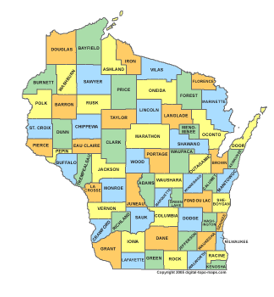## 3. Large- vs Small-Scale

### a. Which is Which?

Which is the larger scale, 1 in = 100 ft  or 1 in = 200 ft? Most people would say 1 in = 200 ft is the larger scale because 200 is greater than 100. That's exactly the wrong logic.
Large and small are distinguished by the drawing size of an object. For example, what size would a 400 ft x 300 ft parcel be on 1 in = 100 ft and 1 in = 200 ft maps?
1 in = 100 ft map

L = 400 ft x (1 in / 100 ft) = 4 in
W = 300 ft x (1 in / 100 ft) = 3 in

1 in = 200 ft map

L = 400 ft x (1 in / 200 ft) = 2 in
W = 300 ft x (1 in / 200 ft) = 1.5 in

The two map representations are shown in Figure C-9.1 in = 100 ft 1 in = 200 ft Figure C-8 Scale Comparison

The parcel plots larger at 1 in = 100 ft than at 1 in = 200 ft. That means 1 in = 100 ft is the larger scale.

### b. Relative Terms

Large scale and small scale are relative terms for comparing one scale to another. There is no formal division between the two; it mostly depends on perspective.
Compare the three maps in Figures C-9 and C-10.(a) Wisconsin (b) Grant County Figure C-9 State and County

Figure C-9(b) map is a larger scale than Figure C-9(a) map.(a) Grant County (b) City of Platteville Figure C-10 County and City

Figure C-10(b) map is a larger scale than Figure C-10(a) map.

In one case, the Grant County map is the larger scale, in the other it is the smaller scale. Going further: a subdivision plat within Platteville would be a larger scale than the Figure C-10(b).